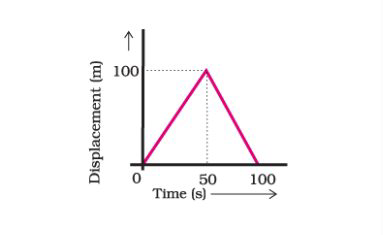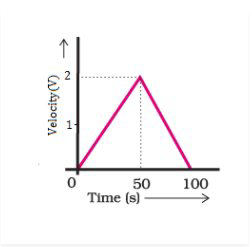# NCERT Exemplar Solutions Class 9 Science Solutions for Motion - Exercise in Chapter 8 - Motion

A girl walks along a straight path to drop a letter in the letterbox and comes back to her initial position. Her displacement–time graph is shown in Fig.8.4. Plot a velocity-time graph for the same.Video transcript
"hi kids welcome to lido india's best live online learning platform so let's see what's the today's question is a girl walks along a straight path to drop a letter in the letterbox and comes back to her initial position her displacement time graph is shown in the figure eight point four plot a velocity time graph for the same so this is the question so what's the question is telling that a girl is there and she is traveling in a straight path to drop a letter and again she comes back to her initial position that means from where she started she came back to the same position so you can see first the graph is increasing and then it is decreasing this is your displacement and here is time so let's try to calculate the velocity for the increasing line right increasing graph increasing means that the velocity is getting greater but what's the formula for the velocity so if you are going to write the formula so velocity is equals to your displacement upon time so for the very first increasing line what's the displacement you can see displacement is 100 and the time taken as 50 so what you will write 50 sorry the displacement is basically 100 not 50. so you will write the displacement value as 100 and time as you can see from here to here it is 50 so you will write 50 and you get the velocity as 2 meter per second for the very first part so here you can see if we want to plot a graph we have taken the velocity and we have taken the time so as the velocity is 2 so we have drawn the velocity 2 and the time is 50. so this graph for the very first half this graph will come like this for the velocity time and now what happens the velocity starts decreasing in this graph what happens your displacement time graph is showing a decrement over here the graph is go going down and then it is becoming complete 0 so now let's try to solve this part again your displacement will be 100 and from 50 to 100 the time is going to be 50 again you will get 2 but this is getting decreased so ultimately it will come in the negative cartesian plane so what will happen again you will mark minus 2 and again you will take the time as what 50 from 50 to 100 so if you are going to plot you will get this particular velocity time graph and here you can see it is minus 2 so you just took the minus 2 part and for 50th it is the 50th second and here you have drawn the 100 second and you simply just joined whole and ultimately you got this graph as an answer so kids this is it for today and if you have any doubts please drop it in the comment section below and do subscribe to leader happy learning "
Related Questions
Exercises

Lido

Courses

Teachers

Book a Demo with us

Syllabus

Maths
CBSE
Maths
ICSE
Science
CBSE

Science
ICSE
English
CBSE
English
ICSE
Coding

Terms & Policies

Selina Question Bank

Maths
Physics
Biology

Allied Question Bank

Chemistry
Connect with us on social media!